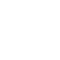# Lap and Wave Winding of DC Machine – Armature Winding Design

In general the lap and wave winding consists of a number of coils connected in series and number of such series circuits are connected in parallel. The coils are diamond shaped and are made in special forming machines. The coils may be single turn or multi turn coil, and a turn consists of two conductors.

The coils are placed in the slots on the armature periphery. In full pitched winding the two coil sides of a coil are placed one pole pitch apart. The de machine armature windings are double layer windings, which means that each slot has two coil sides.

The design of armature winding involves the selection of type of winding, estimation of number of armature coils, turns per coil, conductors per slot, total number of armature conductors and dimensions of the conductor.

#### Types of armature winding

DC machines employ two general types of double layer windings. They are

1. Simplex lap winding
2. Simplex wave winding

These two types of windings primarily differ from each other in the following two factors.

• The number of circuits between the positive and negative brushes, number of parallel paths.
• The manner in which the coil ends are connected to the commutator segments.

In simplex lap winding the number of parallel paths is equal to number of poles, whereas in simplex wave winding the number of parallel paths is two.

In simplex lap winding the finish of a coil is connected to start of next coil. In simplex wave winding the finish of a coil is connected to start of a coil which is lying one pitch away from the finish.

The simplex lap or wave windings are suitable for most of the de machines used for various applications. But occasionally the number of parallel paths have to be increased to a value more than that provided by simplex windings. In such cases the multiplex windings are employed. When the number of parallel paths in a multiplex winding is twice that of simplex winding it is called duplex winding. When the number of parallel paths in multiplex winding is thrice that of simplex winding it is called triplex winding and so on.

In general the lap winding and wave winding refers to simplex windings. The discussions and design aspects presented in this book refers to simplex windings. A comparative study of lap and wave winding is presented in table.

#### Comparison of lap and wave winding

 Lap winding Wave winding The number of parallel paths is equal to number of poles. The number of parallel paths is two. Current through a conductor is l/p, where I, is armature current and p is number of poles. Current through a conductor is 1/2 where l is armature current. For a specified voltage rating, the number of armature conductors required is p/2 times that of wave winding. For a specified voltage rating, the number of armature conductors required is 2/p times that of lap winding. For a specified current rating the area of cross-section of conductor is 2/p times that of wave winding. For a specified current rating the area of cross-section of conductor is p/2 times that of lap winding. For a specified power rating large number of conductors with smaller area of cross section is required. But the volume of copper is same as that of wave winding. For a specified power rating less number of conductors with larger area of cross section is required. But the volume of copper is same as that of lap winding. Since the lap winding has large number of conductors, the area required for insulation is more and so slot area will be large. Also the number of coils will be large. Therefore the cost will be high. Since the wave winding has less number of conductors, the area required for insulation is less and so slot area required will be lesser. Also the number of coils will be less. will be high. Equalizer connections have to be employed. Equalizer connections are not needed. The winding is easier and short pitched coils can be made, which results in reduction in overhang length. It is difficult to wind wave winding and short pitched coils can’t be used. The lap winding is used for large capacity machines and when the current rating is more than 400A. The wave winding is used for small and medium capacity machines. Also used for high voltage and slow speed machines.